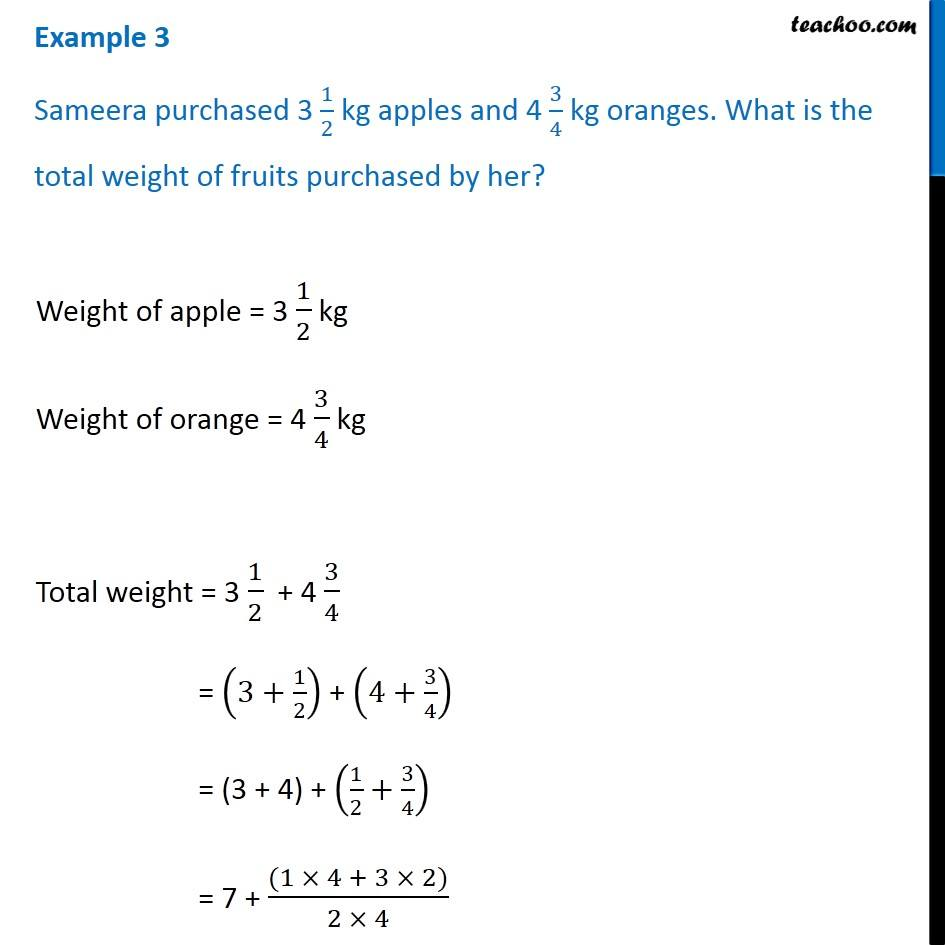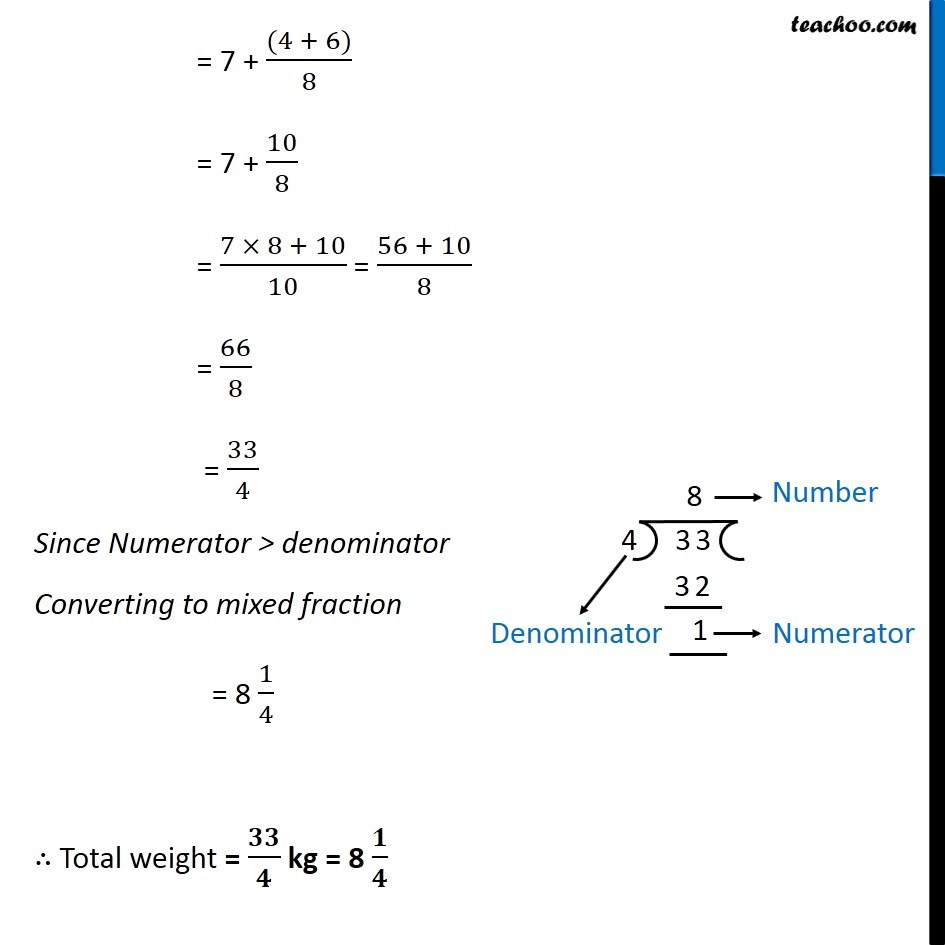Addition and Subtraction of Fractions - Statement Questions

Chapter 2 Class 7 Fractions and Decimals
Concept wiseIntroducing your new favourite teacher - Teachoo Black, at only ₹83 per month

### Transcript

Example 3 Sameera purchased 3 1/2 kg apples and 4 3/4 kg oranges. What is the total weight of fruits purchased by her?Weight of apple = 3 1/2 kg Weight of orange = 4 3/4 kg Total weight = 3 1/2 + 4 3/4 = (3+1/2) + (4+3/4) = (3 + 4) + (1/2+3/4) = 7 + ((1 × 4 + 3 × 2))/(2 × 4) = 7 + ((4 + 6))/8 = 7 + 10/8 = (7 × 8 + 10)/10 = (56 + 10)/8 = 66/8 = 33/4 Since Numerator > denominator Converting to mixed fraction = 8 1/4 ∴ Total weight = 𝟑𝟑/𝟒 kg = 8 𝟏/𝟒